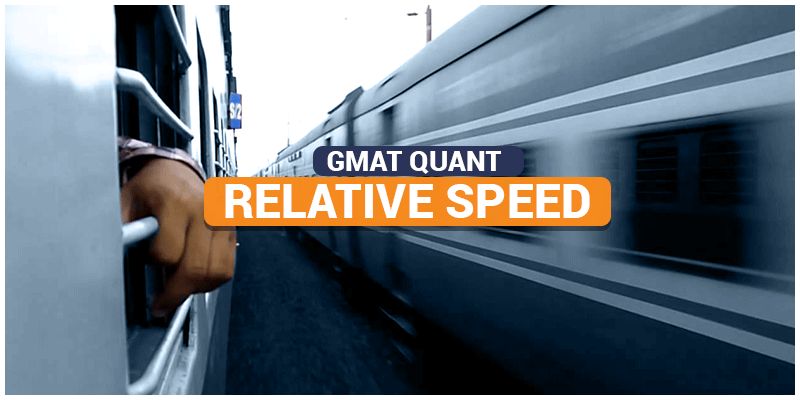# GMAT Quant: Relative SpeedThe concept of relative speed is used to analyze the situation when two or more objects are in motion. In GMAT, there won’t be any questions based on two-dimensional motion as it may require the concept of vectors. Hence, one needs only to focus on the one-dimensional motion.

Let’s say, if two objects are moving in the same direction with speed v2 and v1 from north to south, where v2>v1, then the relative speed will be v2-v1 in the direction from north to south. To infer this, you can imagine an analogous situation, in which the object with speed v1 is kept at rest, and the other object is moving with the speed of v2-v1.

Similarly, if two objects are moving in opposite direction with speed v2 and v1 approaching each other, where v2>v1, then the relative speed will be v2+v1 approaching each other. To infer this, you can imagine an analogous situation, in which the object with speed v1 is kept at rest, and the other object is approaching with the speed of v2+v1.

## Sample Questions on Relative Speed

1. Train ‘A’ of length 80m traveling with the speed of 50m/s overtakes another Train ‘B’ of length 100m traveling with the speed of 30m/s. Find the time was taken to overtake the slower train.

To overtake the train B, train A has to travel train B’ s length, and itself’s length.

That means, distance travelled is 80 + 100 = 180m.

Since they are moving in the same direction, train B can be put to rest and therefore, train

A will be traveling with relative speed of 50-30 =20m/s

Therefore, time taken for overtaking will be 180/20 = 9 second

2. A and B start running in the opposite direction on an athletic track of 400m with the speed of 2m/s and 3m/s respectively. What will be the distance covered by B by the time they cross each for the tenth time.

Let’s put B on rest. Since they are moving in opposite direction, A will be running with

Speed of 2 +3 = 5m/s.

Therefore from the reference of B, A will cover the track with 5 m/s.

Time taken to cover one round will be 400/5 = 80sec

In 80 sec, A will cross B. To cross 10 times; A will take 800 sec.

Therefore, in 800 sec, they will cross each other 10 times.

Hence, distance covered by B in 800 sec = 3*800 = 2400m.